﻿ 适用于洪水遥感SAR图像的CV分割模型研究

适用于洪水遥感SAR图像的CV分割模型研究A Study on CV Segmentation Model for Flood SAR Images

Abstract: The flood caused great inconvenience to people’s life. In order to resume production and living as soon as possible, obtaining the scope of affected areas and the changes in affected areas in time is very important for disaster analysis and post-disaster reconstruction. Due to the influence of speckle noise and large topographic relief in affected area, the flood remote sensing images detected by Synthetic Aperture Radar (SAR) have the disadvantages of many noise points and uneven gray distribution, and the imaging effect is not good. Therefore, it is difficult to achieve accurate image segmentation results. To solve this problem, in this paper, an improved CV segmentation model based on level set method is proposed to achieve rapid and accurate segmentation of flood remote sensing images. CV segmentation model based on region and edge information is very common in the field of medical image segmentation. It can obtain a more accurate segmentation curve, however, it has some defects such as sensitive to noise and over-relying on regional gray mean. For flood remote sensing images with large amount of noise and uneven gray distribution, CV segmentation model is not effective. In order to reduce the interference of noise in the process of forming the segmentation curve, an integral control is added into the energy function of CV segmentation model in this paper to reduce the influence of noise. In addition, in order to improve the segmentation ability of CV segmentation model on the flood remote sensing images with uneven gray scale, the adaptive dynamic weight method was used to fit the segmentation curve for the pixel points in different regions. The experimental results show that, compared with the traditional CV segmentation model and other image segmentation model, the improved CV segmentation model proposed in this paper has better segmentation accuracy on the flood remote sensing images with strong uneven gray distribution, which proves the effectiveness of the improved CV segmentation model proposed in this paper.

1. 引言

CV分割模型结合图像的边缘信息和区域信息，能一定程度上减少相干斑噪声对图像分割效果的影响，且对图像的拓扑结构的变化具有良好的适应性 。但是对于噪点较多、灰度分布不均匀的洪水SAR图像，传统的CV分割模型依然很难达到理想的分割效果。近年来许多学者对CV分割模型做出了改进。Li Chunming等人  提出的基于图像局部区域的可变灰度拟合的RSF模型，一定程度上解决了CV分割模型对灰度不均匀图像分割效果不尽如人意的问题，但是由于过度依赖局部特征，对噪声依然较敏感。陈星等人  提出的LIFCGI模型结合全局信息进行局部图像拟合，在一定程度上解决了RSF模型对噪声较敏感的问题，但是依然无法出色地完成分割灰度分布不均匀图像的工作。

2. 基于水平集方法的CV分割模型

2.1. 水平集方法

$\frac{\partial C}{\partial t}=V\left(C\right)N$ (1)

$\frac{\partial \varphi }{\partial t}=V\left(C\right)|\nabla \varphi |$ (2)

$k=div\left(\frac{\nabla \varphi }{|\nabla \varphi |}\right)$ (3)

2.2. CV分割模型

CV分割模型利用水平集方法得到的闭合曲线C用于分割图像，且令闭合曲线C内部的区域为目标区域，闭合曲线C外部的区域为背景区域 。为了使闭合曲线C尽可能贴近分割区域的轮廓，在CV分割模型中引入一个能量函数，将闭合曲线C的演化问题转变成一个数学模型中的函数最小化问题 。能量函数表达式如式(4)所示：

$\begin{array}{c}{E}^{cv}\left({c}_{1},{c}_{2},\varphi \right)=\mu \cdot \underset{\Omega }{\int }{\delta }_{\epsilon }\left(\varphi \left(x,y\right)\right)|\nabla \varphi \left(x,y\right)|\text{d}x\text{d}y\\ \text{\hspace{0.17em}}+{\lambda }_{1}\cdot \underset{\Omega }{\int }{|{u}_{0}\left(x,y\right)-{c}_{1}|}^{2}{H}_{\epsilon }\left(\varphi \left(x,y\right)\right)\text{d}x\text{d}y\\ \text{\hspace{0.17em}}+{\lambda }_{2}\cdot \underset{\Omega }{\int }{|{u}_{0}\left(x,y\right)-{c}_{2}|}^{2}\left(1-{H}_{\epsilon }\left(\varphi \left(x,y\right)\right)\right)\text{d}x\text{d}y\end{array}$ (4)

$H\left(z\right)=\left\{\begin{array}{l}1,z\ge 0\\ 0,z<0\end{array}$$\delta \left(z\right)=\frac{\text{d}}{\text{d}z}H\left(z\right)$ (5)

${H}_{\epsilon }\left(z\right)$${\delta }_{\epsilon }\left(z\right)$ 的计算公式如式(6)：

${H}_{\epsilon }\left(z\right)=\frac{1}{2}\left[1+\frac{2}{\pi }\mathrm{arctan}\left(\frac{z}{\epsilon }\right)\right]$${\delta }_{\epsilon }\left(z\right)=\frac{1}{\pi }\frac{\epsilon }{{\epsilon }^{2}+{z}^{2}}$ (6)

${c}_{1}$${c}_{2}$ 分别是目标区域和背景区域的灰度均值， ${c}_{1}$${c}_{2}$ 的计算方法如式(7)：

${c}_{1}=\frac{\underset{C}{\iint }{u}_{0}\left(x,y\right)H\left(\varphi \right)\text{d}x\text{d}y}{\underset{C}{\iint }H\left(\varphi \right)\text{d}x\text{d}y}$${c}_{2}=\frac{\underset{C}{\iint }{u}_{0}\left(x,y\right)\mathrm{1-H}\left(\varphi \right)\text{d}x\text{d}y}{\underset{C}{\iint }\mathrm{1-H}\left(\varphi \right)\text{d}x\text{d}y}$ (7)

3. 适用于洪水遥感图像的改进CV分割模型

$\begin{array}{c}{E}^{CV}\left({c}_{1},{c}_{2},\varphi \right)=\mu \cdot \underset{\Omega }{\int }{\delta }_{\epsilon }\left(\varphi \left(x,y\right)\right)|\nabla \varphi \left(x,y\right)|\text{d}x\text{d}y+{\lambda }_{1}\cdot \underset{\Omega }{\int }{|{u}_{0}\left(x,y\right)-{c}_{1}|}^{2}{H}_{\epsilon }\left(\varphi \left(x,y\right)\right)\text{d}x\text{d}y\\ \text{\hspace{0.17em}}+{\lambda }_{2}\cdot \underset{\Omega }{\int }{|{u}_{0}\left(x,y\right)-{c}_{2}|}^{2}\left(1-{H}_{\epsilon }\left(\varphi \left(x,y\right)\right)\right)\text{d}x\text{d}y+{\lambda }_{3}{\left({c}_{1}+{c}_{2}\right)}^{2}\end{array}$ (8)

$\begin{array}{c}{E}^{cv}\left({c}_{1},{c}_{2},\varphi \right)=\mu \cdot \underset{\Omega }{\int }{\delta }_{\epsilon }\left(\varphi \left(x,y\right)\right)|\nabla \varphi \left(x,y\right)|\text{d}x\text{d}y+\frac{{c}_{1}}{{c}_{1}+{c}_{2}}\cdot \underset{\Omega }{\int }{|{u}_{0}\left(x,y\right)-{c}_{1}|}^{2}{H}_{\epsilon }\left(\varphi \left(x,y\right)\right)\text{d}x\text{d}y\\ \text{\hspace{0.17em}}+\frac{{c}_{2}}{{c}_{1}+{c}_{2}}\cdot \underset{\Omega }{\int }{|{u}_{0}\left(x,y\right)-{c}_{2}|}^{2}\left(1-{H}_{\epsilon }\left(\varphi \left(x,y\right)\right)\right)\text{d}x\text{d}y+{\lambda }_{\text{3}}\cdot {\left({c}_{1}+{c}_{2}\right)}^{2}\end{array}$ (9)

$\frac{\partial \varphi }{\partial t}=-\delta \left(\varphi \right)\left[\mu div\left(\frac{\nabla \varphi }{|\nabla \varphi |}\right)-\frac{{c}_{1}}{{c}_{1}+{c}_{2}}{\left({u}_{0}-{c}_{1}\right)}^{2}+\frac{{c}_{2}}{{c}_{1}+{c}_{2}}{\left({u}_{0}-{c}_{2}\right)}^{2}+{\lambda }_{3}{\left({c}_{1}+{c}_{2}\right)}^{2}\right]$

$\varphi \left(x,y,0\right)={\varphi }_{0}\left(x,y\right)$ (10)

$\frac{\partial \varphi }{\partial t}<1{e}^{-5}$ (11)

1) 根据像素点获得水平集函数 $\varphi \left(x,y,t\right)$

2) 初始化水平集函数 $\varphi \left(x,y,t\right)=0$，获得闭合曲线C；

3) 计算目标区域灰度均值 ${c}_{1}$ 和背景区域灰度均值 ${c}_{2}$，计算水平集函数 $\varphi \left(x,y,t\right)=0$ 的梯度值 $\nabla$ 和散度值div；

4) 将 ${c}_{1}$${c}_{2}$$\nabla$ 和div代入加入整体控制项和自适应动态权值的能量函数的梯度方程中，计算并判断能量函数的梯度值是否满足式(11)，从而判定能量函数是否收敛到最小值；

5) 若能量函数没有收敛到最小值，曲线演化到下一个像素点，重新对水平集函数进行初始化，重复以上步骤，直到能量函数达到最小值；

6) 当能量函数达到最小值后，利用Kappa系数  判断分割图像是否精确。Kappa系数的计算公式为式(12)所示：

$\text{Kappa}=\frac{{P}_{0}-{P}_{c}}{1-{P}_{c}}$ (12)

4. 实验结果与分析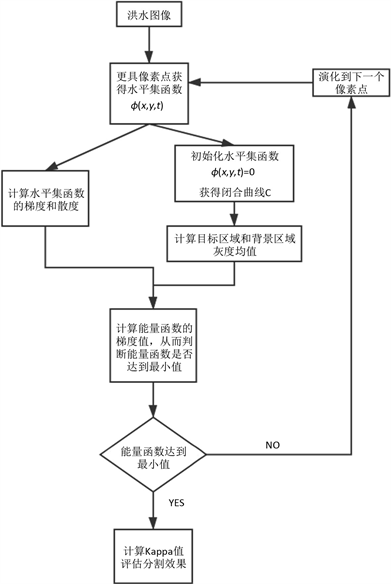Figure 1. Overall flow chart of the improved CV segmentation model for flood remote sensing images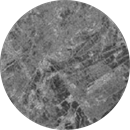(a)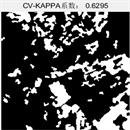(b)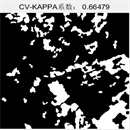(c)(d)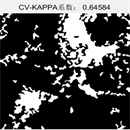(e)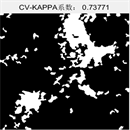(f)

Figure 2. Comparison diagram of detection effect between the traditional CV model and the improved CV model in this paperTable 1. Kappa values of two flood maps compared by traditional CV model and improved CV model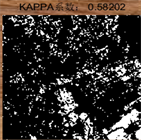(a)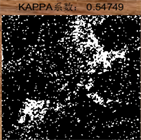(b)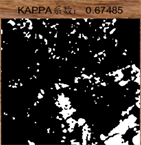(c)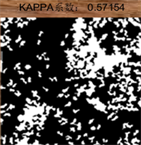(d)(e)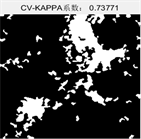(f)

Figure 3. Comparison diagram of detection effect between the improved CV model in this paper and other common image segmentation modelsTable 2. The Kappa value and running time of Indonesia flood map compared by the three modelsTable 3. The Kappa value and running time of the flood map of Java Island in Semarang compared by the three models

5. 结论与展望

 苏慧君. SAR图像分辨率增强方法研究[D]: [硕士学位论文]. 成都: 电子科技大学, 2011: 1-2

 齐小祥, 李敏, 朱颖, 宋雨, 杜卫东. 基于边缘检测的SAR图像自适应区域分割[J]. 计算机工程与应用, 2020(11): 3

 王小丫. 图像分割算法研究与展望[J]. 科技传播, 2019, 11(8): 165-166

 杨晨晓, 辛月兰. 图割综述[J]. 电子设计工程, 2018, 26(6): 60-63.

 许四祥, 李天甲, 翟健健, 等. 基于Prewitt算子的自适应弱小目标检测[J]. 红外技术, 2019, 41(2): 93-97.

 胡高珍. 结合边缘约束的马尔可夫随机场图像分割方法[J]. 电脑知识与技术, 2018, 14(20): 174, 178

 洪向共, 周世芬. 基于灰度共生矩阵和区域生长算法的红外光伏面板图像分割[J]. 科学技术与工程, 2018, 18(34): 92-97

 林挺强, 高峰, 唐沐恩, 文贡坚. 一种新的基于CV模型的图像分割算法[J]. 信号处理, 2010, 26(12): 1852-1857.

 Li, C.M., Kao, C.-Y., Gore, J.C., Ding, Z.H. (2008) Minimization of Region-Scalable Fitting Energy for Image Segmentation. IEEE Transactions on Image Processing, 17, 1940-1949.
https://doi.org/10.1109/TIP.2008.2002304

 陈星, 王艳, 吴漩. 结合全局信息的局部图像灰度拟合模型[J]. 计算机应用, 2018, 38(12): 3574-3579.

 Khosravanian, A., Rahmanimanesh, M., Keshavarzi, P. and Mozaffari, S. (2021) A Level Set Method Based on Domain Transformation and Bias Correction for MRI Brain Tumor Segmentation. Journal of Neuroscience Methods, 352, Article ID: 109091.
https://doi.org/10.1016/j.jneumeth.2021.109091

 林颖, 印桂生, 杨耕. 多相位水平集高分辨率遥感影像非监督分类[J]. 测绘通报, 2009(9): 22-25, 35.

 王瑞花, 宋建社, 高江, 王晓梅. 基于水平集的SAR图像建筑物区域检测[J]. 计算机工程, 2012, 38(12): 191-193.

 李豪. 基于水平集的动态轮廓模型及其在图像分割中的应用[D]: [硕士学位论文]. 西安: 西安电子科技大学.

 高倩倩, 孙世春. 基于CV模型的肝脏核磁共振序列图像自动分割方法[J]. 中国医疗设备, 2020, 35(8): 64-66, 92.

 兰红, 韩纪东. 基于CV模型改进的磁共振成像图像分割方法[J]. 科学技术与工程, 2018, 18(28): 229-234

 Jiang, Y., Dong, J., Nie, D.F. and Zhang, X.Q. (2021) XFEM with Partial Heaviside Function Enrichment for Fracture Analysis. Engineering Fracture Mechanics, 241, Article ID: 107375.
https://doi.org/10.1016/j.engfracmech.2020.107375

 周月娥, 杨绿峰, 李红豫. 基于Dirac函数原理的箱型梁剪力滞效应分析[J]. 广西大学学报(自然科学版), 2020, 45(6): 1324-1331.

 张爱华, 王帆, 陈海燕. 基于改进CV模型的目标多色彩图像分割[J]. 华中科技大学学报, 2018, 46(1): 63-66, 86.

 Zhang, Z.X., Jiang, M.R., Zhang, J., Zhang, K.G. and Miao, Y. (2011) Meteorological Objects Segmentation Based on Improved CV Model. Pro-ceedings of 2011 World Congress on Engineering and Technology, Shanghai, 28 October 2011, Vol. 7, 78-83.

 von Below, J. and Kaul, H. (2021) A Variational Approach to Relativistic Fluid Balls. Calculus of Varia-tions and Partial Differential Equations, 60, Article No. 31.
https://doi.org/10.1007/s00526-020-01898-z

 王娇, 方全, 罗芬, 张美玲, 唐奇伶. 融合双眼特征的糖网病图像识别方法[J]. 中南民族大学学报(自然科学版), 2020, 39(6): 607-613.

 李春, 刘朝霞. 一个改进的CV活动轮廓模型[J]. 中央民族大学学报(自然科学版), 2014, 23(4): 83-87.

 苏慧君. SAR图像分辨率增强方法研究[D]: [硕士学位论文]. 成都: 电子科技大学, 2011: 1-2

 齐小祥, 李敏, 朱颖, 宋雨, 杜卫东. 基于边缘检测的SAR图像自适应区域分割[J]. 计算机工程与应用, 2020(11): 3

 王小丫. 图像分割算法研究与展望[J]. 科技传播, 2019, 11(8): 165-166

 杨晨晓, 辛月兰. 图割综述[J]. 电子设计工程, 2018, 26(6): 60-63.

 许四祥, 李天甲, 翟健健, 等. 基于Prewitt算子的自适应弱小目标检测[J]. 红外技术, 2019, 41(2): 93-97.

 胡高珍. 结合边缘约束的马尔可夫随机场图像分割方法[J]. 电脑知识与技术, 2018, 14(20): 174, 178

 洪向共, 周世芬. 基于灰度共生矩阵和区域生长算法的红外光伏面板图像分割[J]. 科学技术与工程, 2018, 18(34): 92-97

 林挺强, 高峰, 唐沐恩, 文贡坚. 一种新的基于CV模型的图像分割算法[J]. 信号处理, 2010, 26(12): 1852-1857.

 Li, C.M., Kao, C.-Y., Gore, J.C., Ding, Z.H. (2008) Minimization of Region-Scalable Fitting Energy for Image Segmentation. IEEE Transactions on Image Processing, 17, 1940-1949.
https://doi.org/10.1109/TIP.2008.2002304

 陈星, 王艳, 吴漩. 结合全局信息的局部图像灰度拟合模型[J]. 计算机应用, 2018, 38(12): 3574-3579.

 Khosravanian, A., Rahmanimanesh, M., Keshavarzi, P. and Mozaffari, S. (2021) A Level Set Method Based on Domain Transformation and Bias Correction for MRI Brain Tumor Segmentation. Journal of Neuroscience Methods, 352, Article ID: 109091.
https://doi.org/10.1016/j.jneumeth.2021.109091

 林颖, 印桂生, 杨耕. 多相位水平集高分辨率遥感影像非监督分类[J]. 测绘通报, 2009(9): 22-25, 35.

 王瑞花, 宋建社, 高江, 王晓梅. 基于水平集的SAR图像建筑物区域检测[J]. 计算机工程, 2012, 38(12): 191-193.

 李豪. 基于水平集的动态轮廓模型及其在图像分割中的应用[D]: [硕士学位论文]. 西安: 西安电子科技大学.

 高倩倩, 孙世春. 基于CV模型的肝脏核磁共振序列图像自动分割方法[J]. 中国医疗设备, 2020, 35(8): 64-66, 92.

 兰红, 韩纪东. 基于CV模型改进的磁共振成像图像分割方法[J]. 科学技术与工程, 2018, 18(28): 229-234

 Jiang, Y., Dong, J., Nie, D.F. and Zhang, X.Q. (2021) XFEM with Partial Heaviside Function Enrichment for Fracture Analysis. Engineering Fracture Mechanics, 241, Article ID: 107375.
https://doi.org/10.1016/j.engfracmech.2020.107375

 周月娥, 杨绿峰, 李红豫. 基于Dirac函数原理的箱型梁剪力滞效应分析[J]. 广西大学学报(自然科学版), 2020, 45(6): 1324-1331.

 张爱华, 王帆, 陈海燕. 基于改进CV模型的目标多色彩图像分割[J]. 华中科技大学学报, 2018, 46(1): 63-66, 86.

 Zhang, Z.X., Jiang, M.R., Zhang, J., Zhang, K.G. and Miao, Y. (2011) Meteorological Objects Segmentation Based on Improved CV Model. Pro-ceedings of 2011 World Congress on Engineering and Technology, Shanghai, 28 October 2011, Vol. 7, 78-83.

 von Below, J. and Kaul, H. (2021) A Variational Approach to Relativistic Fluid Balls. Calculus of Varia-tions and Partial Differential Equations, 60, Article No. 31.
https://doi.org/10.1007/s00526-020-01898-z

 王娇, 方全, 罗芬, 张美玲, 唐奇伶. 融合双眼特征的糖网病图像识别方法[J]. 中南民族大学学报(自然科学版), 2020, 39(6): 607-613.

 李春, 刘朝霞. 一个改进的CV活动轮廓模型[J]. 中央民族大学学报(自然科学版), 2014, 23(4): 83-87.

Top